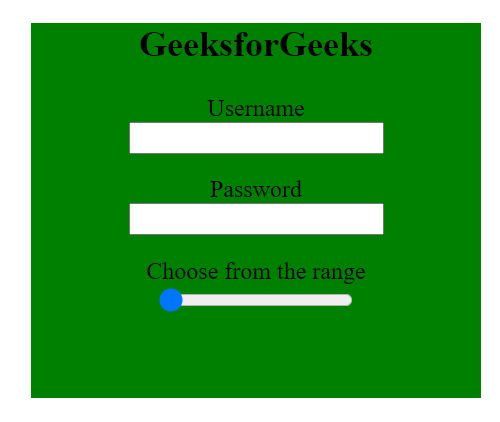GeeksforGeeks App
Open AppBrowser
Continue

# Which specific tag is used for describing the range of numbers in HTML Form ?

The HTML input tag is used for describing the range of numbers in HTML form. The <input> tag is used to define an input field where users enter data. It is used within <form> elements. The input format for the user can be of various types depending on the value of the type attribute of <input> tag.

Describing the range of numbers in HTML Form : The <input> type attribute specifies the type of <input> element to display. “text” is the default value of type attribute of<input>. For describing the range of numbers, the value of type attribute of <input> element is “range“.

Syntax:

`<input type = "range" > `

It defines the control for a number entered by the user whose exact value is not important( more like a slider control).  Its default range varies from 0 to 100.

Restrictions can be set on what numbers are accepted by using additional attributes such as:

• step – it specifies the legal number intervals, the default value is 1
• value – it specifies the default value
• max – it specifies the maximum value allowed. The default is 100
• min – it specifies the minimum value allowed, the default is 0

Note: If range type is not supported by the user’s browser, it will be treated as text input.

Example :

In this example <input type=”range”> is used for describing range . The user can choose from values 1 to 10 by increasing a step of value 2, by default value kept here is 1.

## HTML

 ```<``html``>`` ` `<``head``>``    ``<``title``>Describing Range of Numbers in HTML Form``    ``<``style``>``        ``.container {``            ``height: 250px;``            ``width: 300px;``            ``background-color: green;``            ``text-align: center;``            ``margin: auto;``        ``}``         ` `        ``.container div {``            ``margin-top: 15px;``        ``}``    `````` ` `<``body``>`` ` `    ``<``div` `class``=``"container"``>``        ``<``h2``>GeeksforGeeks``        ``<``form``>``            ``<``div``>``                ``<``label` `for``=``"username"``>``                  ``Username``                ``<``br``>``                ``<``input` `type``=``"text"``                       ``id``=``"username"` `                       ``name``=``"username"``>``            ````            ``<``div``>``                ``<``label` `for``=``"password"``>``                  ``Password``                ``<``br``>``                ``<``input` `type``=``"password"` `                       ``id``=``"password"` `                       ``name``=``"password"``>``            ````            ``<``div``>``                ``<``label` `for``=``"gfg_range"``>``                  ``Choose from the range ``                ````                ``<``br``>``                ``<``input` `type``=``"range"` `                       ``id``=``"gfg_range"` `                       ``min``=``"1"` `                       ``value``=``"1"` `                       ``max``=``"10"` `                       ``step``=``"2"``>``            ````        ````    ```` ` ` ` ``` ` ``

Output :My Personal Notes arrow_drop_up
Related Tutorials